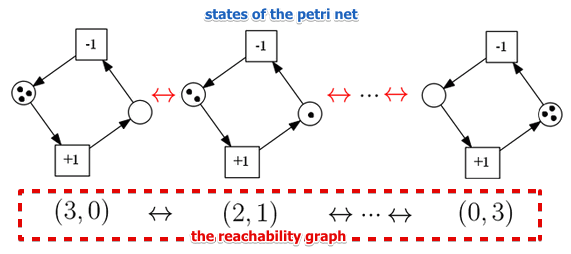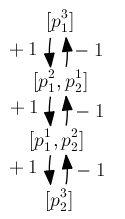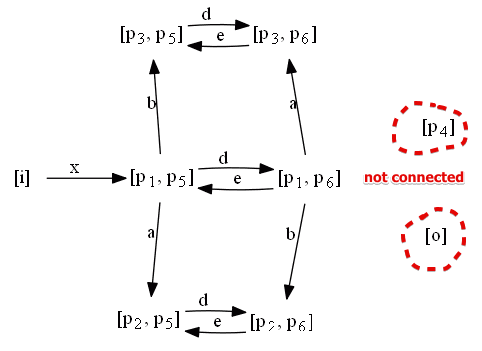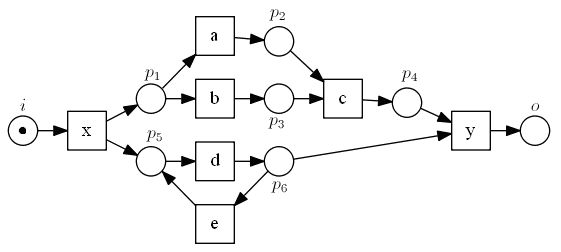# ML Wiki

## Reachability Graph

This is a way of representing the states of Petri Nets

A marking $M$ is reachable from the initial marking $M_0$

• $\iff M_0 \to^* M$
• i.e. there exists a firing sequence that brings us from the initial state of a petri net to a state that corresponds to $M_0$

In a reachability graph of a petri net $N = (P, T, F)$

• nodes correspond to reachable markings
• edges correspond to the relation $\to$

There can be several notations for markings:

• first one: $(n_1, n_2, ..., n_m)$ corresponds to the number of elements in the places $(p_1, ..., p_m)$ respectively
• second one: $[p_1^{n_1}, ..., p_m^{n_m}]$ where $n_i$ is the number of times $p_i$ appears
• if $p_i$ appears zero times, we don't show it in the marking

Consider this example:

•• so a reachability graph shows all possible states (markings) that you can reach by triggering transitions that are enabled
•• the graph above is the reachability graph with different notation

There are some desirable properties for the Workflow Nets

• we want to be able to decide on them
• reachability graphs can be used for that

### Coverability Graph

This is a similar notion for expressing states of Workflow Nets

• but unlike Reachability Graph it can be finite for unbounded nets
• yet in many cases less expressive

## Examples

### Example 1

•• it is a reachability graph for
•• we see that two nodes are not connected: it's clearly a problem